Get instant live expert help with Excel or Google Sheets“My Excelchat expert helped me in less than 20 minutes, saving me what would have been 5 hours of work!”

#### Post your problem and you’ll get expert help in seconds.

Your message must be at least 40 characters
Our professional experts are available now. Your privacy is guaranteed.

# Several Ways to Calculate the Internal Rate of Return in Excel

Excel allows a user to get an internal rate of return of an investment in several ways, using the IRR, XIRR and MIRR functions. This step by step tutorial will assist all levels of Excel users in learning several ways to calculate the internal rate of return in Excel.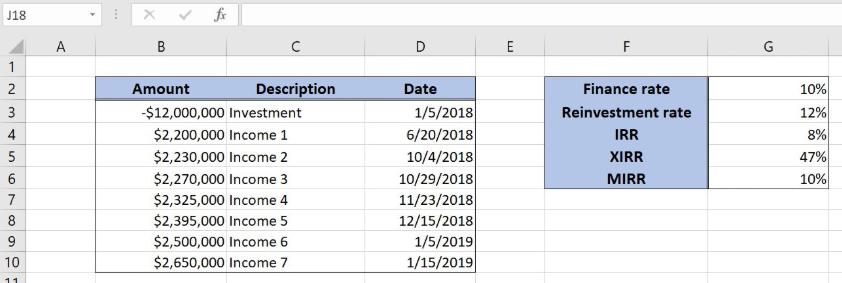Figure 1. The result of the IRR, XIRR and MIRR functions

## Syntax of the IRR Formula

The generic formula for the IRR function is:

`=IRR (values, [guess])`

The parameter of the IRR function is:

• values – a range of cells containing values, including initial investment and incomes. The investment must have negative sign, as it is a cost
• [guess] – an estimated value for the expected IRR. This parameter is non-mandatory. If it’s omitted, the function will take a default value of 0.1 (=10%).

## Syntax of the XIRR Formula

The generic formula for the XIRR function is:

`=XIRR (values, dates, [guess])`

The parameter of the XIRR function is:

• values – a range of cells containing values, including initial investment and incomes. The investment must have negative sign, as it is a cost
• dates – a range of dates corresponding to values. All dates must be in chronological order
• [guess] – an estimated value for the expected XIRR. This parameter is non-mandatory. If it’s omitted, the function will take a default value of 0.1 (=10%).

## Syntax of the MIRR Formula

The generic formula for the MIRR function is:

`=MIRR (values, [guess])`

The parameter of the MIRR function is:

• values – a range of cells containing values, including initial investment and incomes. The investment must have negative sign, as it is a cost
• finance_rate – a discount rate of the investment returns
• reinvest_rate – an interest rate for reinvested returns.

## Setting up Our Data for the Functions

Let’s look at the structure of the data we will use. In column B (“Amount”), we have values including initial investment and yearly incomes. In column C (“Description”) we have description of every amount. In column D (“Date”), we have dates. In In the cell G4, G5 and G6, we want to get the IRR, XIRR and MIRR respectively.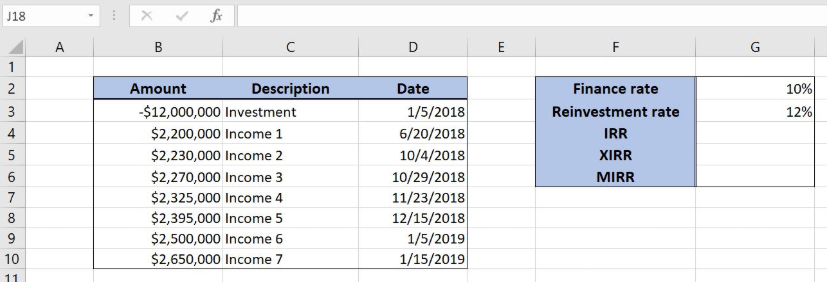Figure 2. Data that we will use in the examples

## Get an IRR of Values Using the IRR Function

In our example, we want to get the IRR of the values in the range B3:B10. The result will be in the cell E3.

The formula looks like:

`=IRR(B3:B10)`

The parameter values is the range B3:B10.

To apply the IRR function, we need to follow these steps:

• Select cell G4 and click on it
• Insert the formula: `=IRR(B3:B10)`
• Press enter.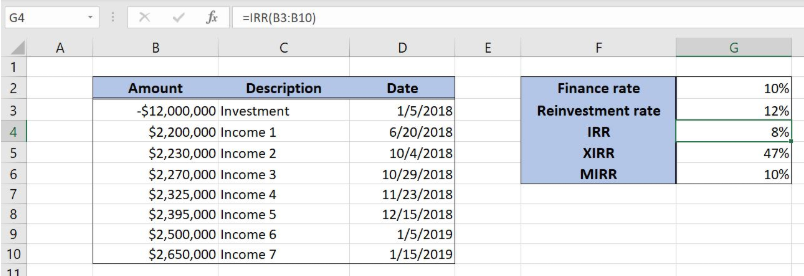Figure 3. Using the IRR function to get the internal rate of the investment

Finally, the result in the cell G4 is 8%, which is the internal rate of the investment using the IRR function.

## Get an XIRR of Values Using the XIRR Function

In our example, we want to get the XIRR of the values in the range B3:B10. The result will be in the cell E3.

The formula looks like:

`=XIRR(B3:B10, D3:D10)`

The parameter values is the range B3:B10 and the parameter dates is D3:D10.

To apply the XIRR function, we need to follow these steps:

• Select cell G5 and click on it
• Insert the formula: `=XIRR(B3:B10, D3:D10)`
• Press enter.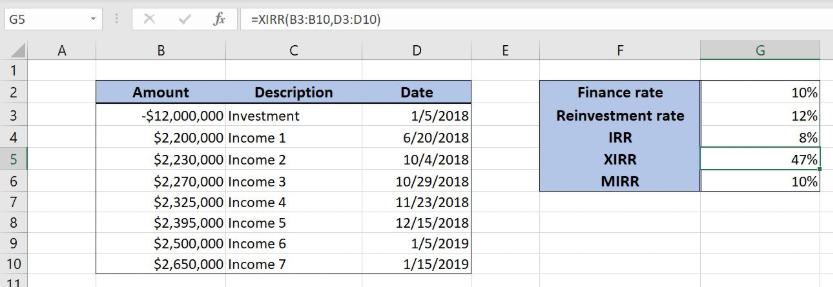Figure 4. Using the XIRR function to get the internal rate of the investment

Finally, the result in the cell G5 is 47%, which is the internal rate of the investment using the XIRR function.

## Get a MIRR of Values Using the MIRR Function

In our example, we want to get the MIRR of the values in the range B3:B10. The finance rate is 10% (G2) and the reinvestment rate is 12% (G3). The result will be in the cell F4.

The formula looks like:

`=MIRR(B3:B10, G2, G3)`

The parameter values is the range B3:B10. The finance_rate is in G2, while the reinvest_rate is in G3.

To apply the MIRR function, we need to follow these steps:

• Select cell G6 and click on it
• Insert the formula: `=MIRR(B3:B10, G2, G3)`
• Press enter.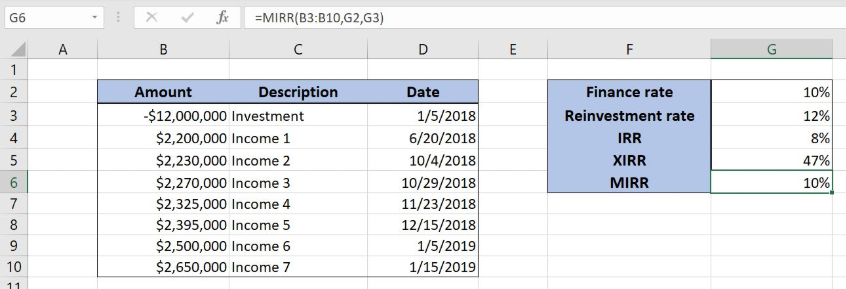Figure 5. Using the MIRR function to get the modified internal rate of the investment

Finally, the result in the cell G6 is 10%, which is the modified internal rate of the investment using the MIRR function.

Most of the time, the problem you will need to solve will be more complex than a simple application of a formula or function. If you want to save hours of research and frustration, try our live Excelchat service! Our Excel Experts are available 24/7 to answer any Excel question you may have. We guarantee a connection within 30 seconds and a customized solution within 20 minutes.